# 13 Free Math Worksheets and Activities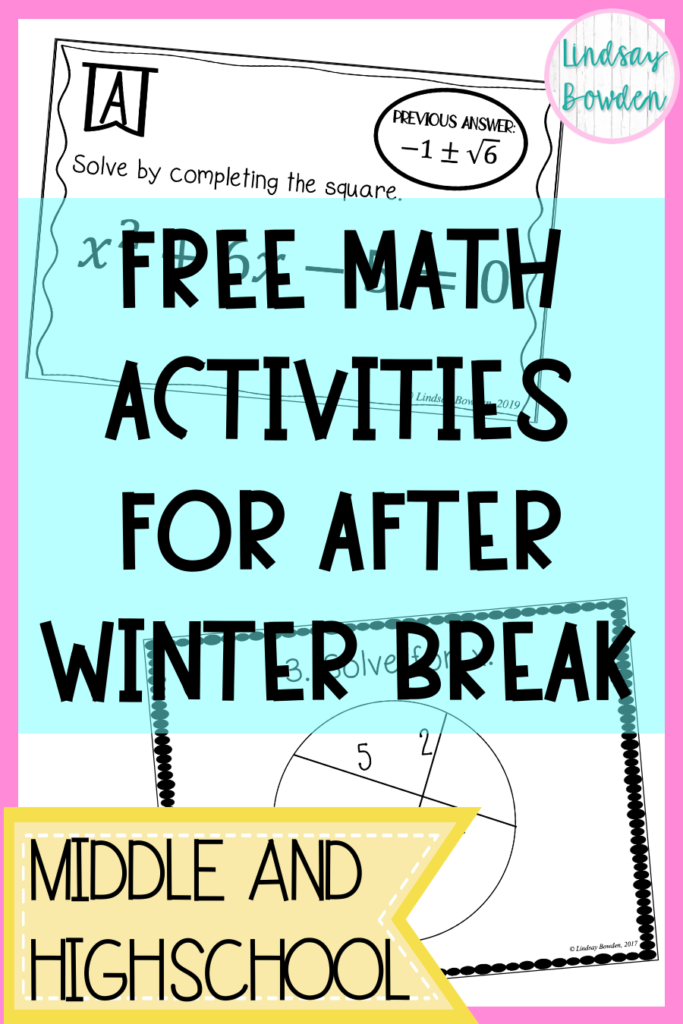Are you looking for a free math worksheets or activities to do with your students after winter break? I’ve got you!

I’m sure you’re tired and maybe not thrilled about being back at work. You definitely don’t want to spend time prepping an activity or lesson from scratch. These activities are fun and engaging. Your students will love them!

## 1. Decimal Operations QR Code Activity (5th-7th grade)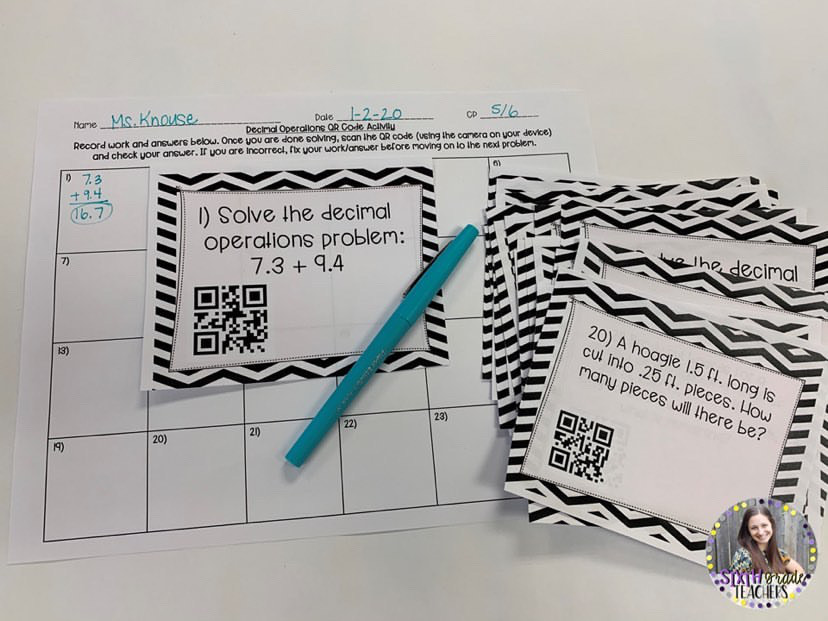This Decimal Operations QR Code Activity from Sixth Grade Teachers is the perfect way to engage students with learning, technology, and group work. This 24 question activity allows students to practice solving addition, subtraction, multiplication, and division problems with decimals.

The activity is designed for students to work in small groups, but students can work individually or in partners. Print the problems for each group or hang around the room and students can start at different spots.

First, students will solve the problem and record work on the recording sheet. Next, using the camera on the device, students will scan the QR code. Then, students should fix answers that are incorrect before moving on to the next question.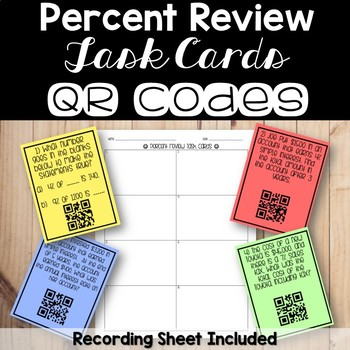In this activity from Count On Me, students answer questions involving percent concepts. Topics include basic percent questions, discounts, tax, tip, and simple interest.

The task cards have real life applications, so it is awesome for students to see how these percent questions can be applied to real world situations.

This activity also has QR codes where students can check their answers.

## 3. Combining Like Terms Activity (6th-8th grade)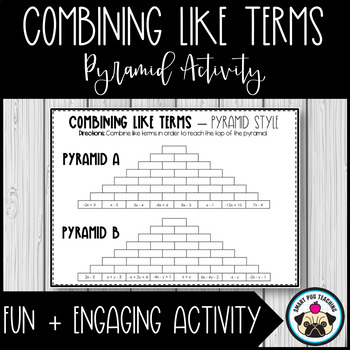In this fun activity from Smart Pug Teaching, students will be assessed on their ability to combine like terms.

Students will begin on the bottom row of the pyramid. They will simplify adjacent expressions in order to identify the next expression. They will continue to simplify until they have reached the top of the pyramid.

Two pyramids and answer keys are provided. There is also a blank copy for students to create their own pyramid!

## 4. One-Step Equations Digital Activity (6th-8th)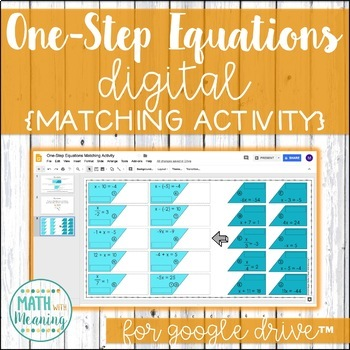If you have access to technology in your classroom, this digital activity by Math with Meaning is perfect! If you’re not 1:1, there is a paper version too.

This matching activity contains problems for students to answer using Google Drive.

Students will solve 20 equations and make matching sets of cards that have the same solution.

The equations include both positive and negative numbers and fractional coefficients.

## 5. Two-Step Equation Activity (6th-8th Grade)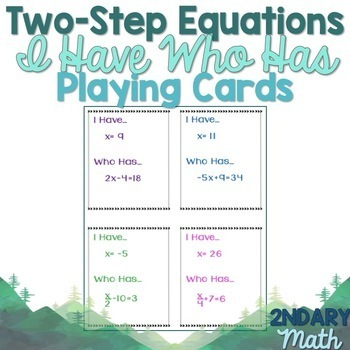In this activity by 2ndary Math, students will solve two-step equations while playing a fun game.

Start by printing and cutting out the cards. Give each student a card. Pick one student to start by reading their “Who has” portion.

Then, the class solves the equation. Whoever has the answer says “I have ___”. The game continues until all the cards have been used.

## 6. Function or Not Activity (8th-9th grade)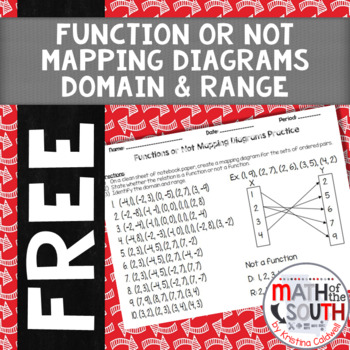This activity by Math of the South is a great review of functions, domain, and range.

This is a practice assignment that can be used to practice creating mapping diagrams for sets of ordered pairs.

The practice directions also ask the students to identify whether the relation is a function and the domain and range. This free math worksheet is the perfect review before beginning new material after winter break. Answer keys are included!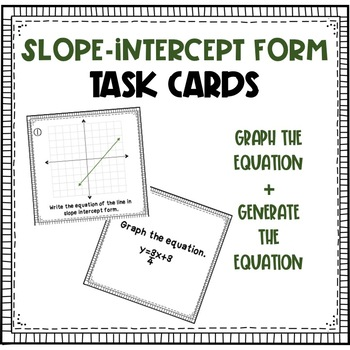Students can always use extra practice on slope-intercept form. These task cards by Brooklyn’s Brightest are a a great way to review linear equations.

There are 12 questions in this activity. Students will be asked to graph the equation and generate the equation. Answer keys are included! If you taught linear equations before winter break, this is a great way to review when you go back!

## 8. Point-Slope Form Graphing Activity (7th-10th)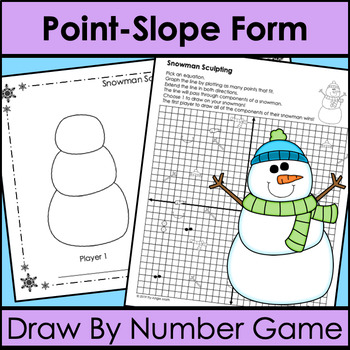This game by Try-Angle Math is a perfect winter activity!

Students pick an equation card and graph the line using point-slope form. Students pick one item that the line passes through to draw on their snowman. The first person to draw a complete snowman wins.

You can split your class into groups of 2-4 to play this game!

## 9. Writing Parallel and Perpendicular Equations Activity (8th-10th grade)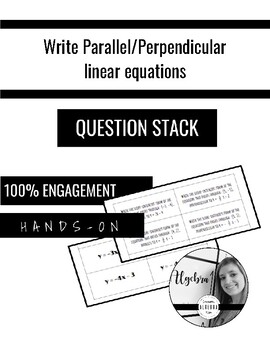This question stack by Damman’s Algebra Room is perfect for Algebra or Geometry!

Question Stack is an engaging, self-checking, loop activity. Students lay all their cards with answers facing up and select one question (directions are on the activity card which they place their stack of cards).

Once the first question is solved, they find the answer and then flip and stack to reveal the next question. This activity helps students practice writing parallel and perpendicular lines. There are 10 questions included.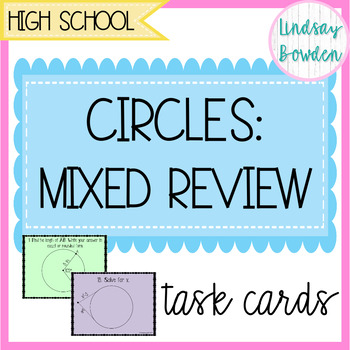Review all the circle theorems in this task cards activity!

• arc length
• measures of angles/arcs involving central, inscribed, vertex inside, and vertex outside angles
• tangent segments and a radius (Pythagorean Theorem)
• segment lengths (two chords, two secants, secant & tangent)

There are 20 questions, a student answer sheet, and answer keys included!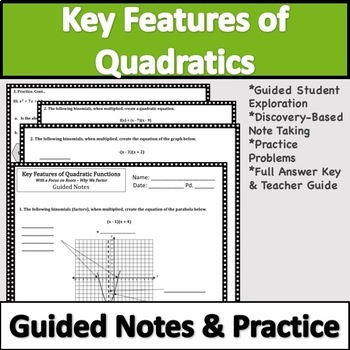Do you need a lesson on quadratic functions? These guided notes and worksheets by Lauren Fulton are exactly what you need!

This  set of guided notes introduces key features of quadratic functions and graphs to your students. Students discover roots, x-intercepts, zeros, solutions, axis of symmetry, y-intercept, vertex, minimums & maximums!

This lesson is a perfect introduction to key features  and transformations of quadratics in vertex or standard form. It could even be used as an introduction to factoring. Answer keys included!

## 12. Converting Logarithmic and Exponential Form Activity (10th-12th)In this activity by Math with Ms. Rivera, students practice converting from logarithmic to exponential form and exponential to logarithmic form. It’s difficult to find free math worksheets and activities for upper level math. You definitely want to grab this freebie!

This activity includes 19 problems that use actual values, natural log, e, and variables. The best part? It’s self-checking! This activity can be used individually or in small groups.

Start by printing and cutting out the pieces. Students will start with the piece that says “Start” on the small end. Then complete the problem on that card. Once students have correctly converted the logarithm or exponential functions, they must find the answer on the small end of another card.

## 13. Goal Setting Worksheet (any grade)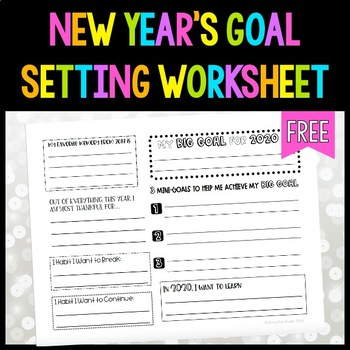Don’t feel like a math activity on your first day back? Busy Miss Beebe has this awesome goal setting activity!

Students will create goals for the year including mini-goals and one big goals. The worksheet also covers habits to continue and break.

## BONUS: Another free math activity!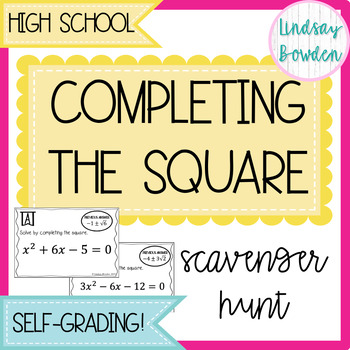Ok I couldn’t just stop at 13. Here is one more free math activity from my store! In this scavenger hunt, students will solve 12 quadratic equations by completing the square.

For all trinomials, a=1. None of them are factorable. The best part about this scavenger hunt? It’s self-grading! If students get back to their original problem, they know they got all problems correct.

I hope you loved these free math worksheets and activities! Let me know in the comments below!

You may also like:

Happy Teaching!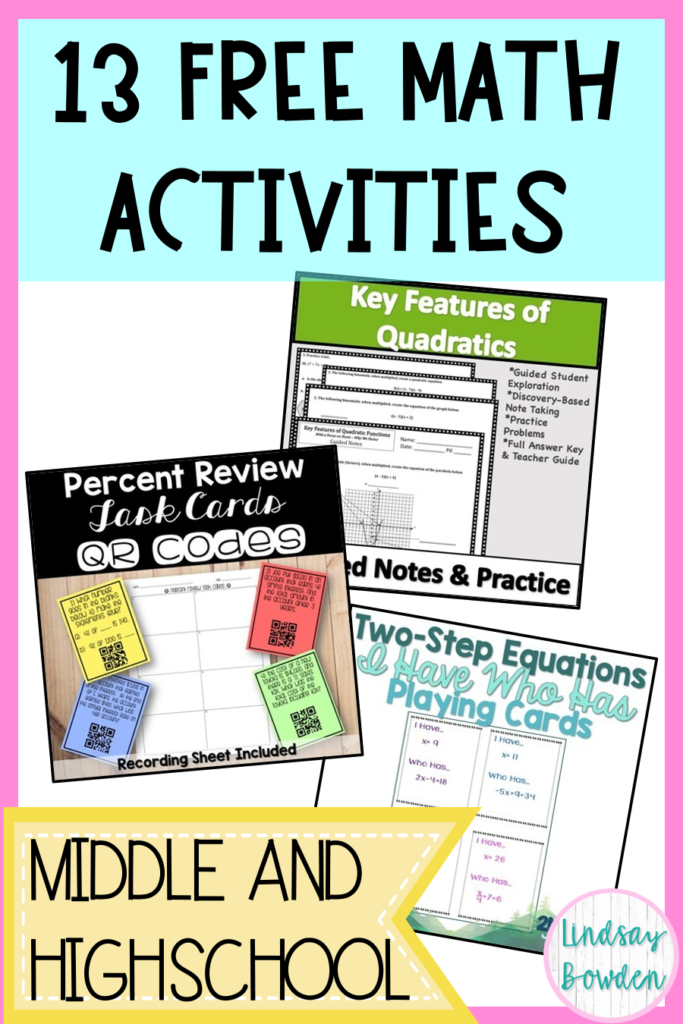## Lindsay